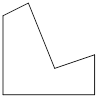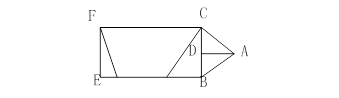### Sample Problem

How many pairs of parallel lines does each figure have?

1)There are pairs of parallel lines.

2)There are pairs of parallel lines.

#### Solution

1) There is 2 pairs of parallel lines in the 1st figure.

2) There are 4 pairs of parallel lines in the 2nd figure.FC|| EB, FE||CB and AD||CF, AD||BE.